# Bus vs. train

The bus started from point A 10 minutes before the train started from the same place. The bus went an average speed of 49 km/h, train 77 km/h.
To point B train and bus arrived simultaneously.

Calculate the time of the train journey, if train and bus traveled the same distance.

Correct result:

t =  17.5 min

#### Solution:

$\ \\ s = v_1 (t+10)/60 = v_2 t/60 \ \\ \ \\ 49 (t+10) = 77 t \ \\ \ \\ t = \dfrac{ 49\cdot 10}{ 77-49 } = 17.5 \ \text{min}$

49 (t+10) = 77 t

49•(t+10) = 77•t

28t = 490

t = 35/2 = 17.5

Our simple equation calculator calculates it.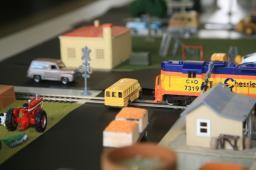We would be pleased if you find an error in the word problem, spelling mistakes, or inaccuracies and send it to us. Thank you!Tips to related online calculators
Do you have a linear equation or system of equations and looking for its solution? Or do you have quadratic equation?
Do you want to convert length units?
Do you want to convert velocity (speed) units?
Do you want to convert time units like minutes to seconds?

## Next similar math problems:

• Two trains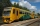From station A, the freight train traveled at a speed of 40 km/h in 9h. When he drove 15km, the fast train started from station A in the same direction at a speed of 70km/h. When will it the freight train catch up?
• Motion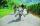Cyclist started at 9:00 from point S to point T. After 10 minutes, followed him at the same speed the second cyclist. Walker, which went from T to S, started at 9:35. After 71 minutes he met the first cyclist and after next 8 minutes the second cyclist. D
• Train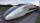A passenger train traveled for 2 hours 74 km. 3.1 hours after its departure started fast train and caught it on 186 km. How many km/h is different its average speeds?
• MotionIf you go at speed 3.7 km/h, you come to the station 42 minutes after leaving the train. If you go by bike to the station at speed 27 km/h, you come to the station 56 minutes before its departure. How far is the train station?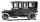Car started at 11:40 at speed 54 km/h from A and to B arrived at 12:20. Determine the distance A and B.
• Chalet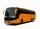The children were on a school trip to the mountain . One third of the journey went by the train and continue by bus. When you get off the bus, they remained a third of the distance they traveled by bus. What part of journey children went by the bus (wrote
• Motion 2At 10 o'clock started from place A car speed at 90 km/h. 20 minutes after started from the place B at speed 60 km/h another car. Distance places A and B is 120 kilometers. What time and where they meet?
• Bus stop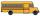Determine at what time the bus leaves from the bus stop outside the village, if you know that when you leave home at 8:00 and go at speed 3 km/h, you come to a stop 9 minutes after the departure of the bus, and when you go speed at 4 km/h, you come to the
• Train speedTwo guns were fired from the same place at an interval of 10 minutes and 30 seconds, but a person in a train approaching the place hears second shot 10 minutes after the first. The speed of the train (in km/hr), supposing that sound travels at 340 m/s is:
• Pedestrian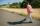Dana started at 10:00 from the point A to point B. These points are distant 12 km. Determine how fast Daniel went when the place B arrived at 11:54. Speed express in km/h.
• Average speedMichal went out of the house by car at speed 98 km/h. He came into the goal place for 270 minutes. Determine the distance between the two places.
• TruckIn 7 hours started from town Krnov truck at speed 40 km/h. Passenger car started against it in 8 hours 30 minutes from the city of Jihlava at speed 70 km/h. Distance between this two cities is 225 km. At what time and at what distance from Krnov this two
• MotionFrom two different locations distant 232 km started against car and bus. The car started at 5:20 with average speed 64 km/h. Bus started at 7:30 with average speed 80 km/h. When they meet? How many kilometers went the bus?
• Warehouse carsFrom the warehouse started truck at speed 40km/h. After 1hour 30mins started from the same place same direction a car at speed 70 km/h. For how long and at what distance from the warehouse overtake a truck?
• Reconstruction of the corridorCalculate how many minutes will be reduced to travel 187 km long railway corridor, where the maximum speed increases from 120 km/h to 160 km/h. Calculate how many minutes will shorten travel time, if we consider that the train must stop at 6 stations, eac
• Two trains meetFrom A started at 7:15 express train at speed 85 km/h to B. From B started passenger train at 8:30 in the direction to A and at speed 55 km/h. The distance A and B are 386 1/4 km. At what time and at what distance from B the two trains meet?
• Forth and back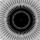Car goes from point A to point B at speed 78 km/h and back at 82 km/h. If went there and back at speed 81 km/h journey would take five minutes less. What is the distance between points A and B?